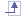# Glossary

## Ccable joint A cable type joint between three products (two products are mobile, the other is a reference). Number of degrees of freedom is 1 (translation).
cylindrical joint A translation type joint between two products along an axis with a rotation about that axis. Number of degrees of freedom is 2 (1 translation and 1 rotation). This joint was called Actuator in Version 4.
command An angular or linear command that drives the kinematics mechanism.
CV joint A constant velocity joint between two products. Number of degrees of freedom is 4 (comprises two U joints).

## Ddegrees of freedom The number of possible independent rotation or translation movements of a joint.
dress up A list of models attached to a set of the kinematics model. These models have the same motion as the set.

## F

fixed product The product that remains stationary when the kinematics mechanism is in motion.

## G

gear joint A gear type joint between three products (two products are pinions, the other is a reference). Number of degrees of freedom is 1 (rotation).

## Jjoint A constraint between geometric entities of two or three products. There are several types of joint.
joint stop An imposed limit applied to a joint.

## K

kinematics mechanism A mechanism comprising several products that are connected by joints.

It can be simulated when the number of commands is equal to degrees of freedom (in this case the mechanism is said to be complete).

kinematics product A rigid product defined in a single geometric set that contains all the elements required to describe the kinematics mechanism and its motion.
kinematics simulation A simulation of the mechanism's motion using commands. Simulation can be immediate (commands are used one by one) or on request (one or more commands are used with a given number of steps).

## L

law A numeric or graphic representation of the commands applied to a kinematics mechanism as a function of time.

## P

planar joint A planar joint between two products. Number of degrees of freedom is 3 (1 rotation and 2 translations).
prismatic joint A translation joint between tow products along an axis with no rotation about that axis. Number of degrees of freedom is 1 (translation).
PT/CRV joint A point/curve joint between two products. Number of degrees of freedom is 4 (3 rotation and 1 translation) for a 3D mechanism and 2 (1 rotation and 1 translation) for a 2D mechanism.
PT/SUR joint A point/surface joint between two products. Number of degrees of freedom is 5 (3 rotations and 2 translations).

## Rrack joint A gear/rack type joint between three products (one product is the rack, another is the rack, the other is a reference). Number of degrees of freedom is 1 (combined translation and rotation).
revolute joint A revolute joint about an axis between two products with no translation along that axis. Number of degrees of freedom is 1 (rotation).
rigid joint A rigid (fully restricted) joint between two products. There are no degrees of freedom associated to this joint.
roll/CRV joint A rolling type joint between two products that include curves. There is no sliding motion with this type of joint. Number of degrees of freedom is 2 (1 rotation and 1 translation) for a 3D mechanism and 1 (translation) for a 2D mechanism.

## S

screw joint A screw/nut type joint between two products relative to an axis. Number of degrees of freedom is 1 (combined translation and rotation).
slid/CRV joint A rolling type joint with a sliding motion between two products that include curves. Number of degrees of freedom is 3 (2 rotations and 1 translation) for a 3D mechanism and 2 (1 rotation and 1 translation) for a 2D mechanism.
spherical joint A spherical joint between two products. Number of degrees of freedom is 3 (3 rotations) for a 3D mechanism and 1 (rotation) for a 2D mechanism. This joint was called PT/PT in Version 4.
storyboard A recorded kinematics motion.

## UU joint A universal joint between two products. Number of degrees of freedom is 2 (2 rotations).